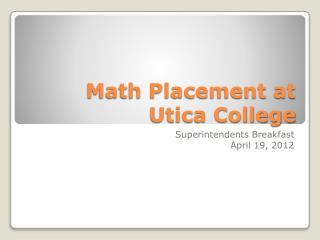# Math Placement at Utica College - PowerPoint PPT PresentationDownload PresentationMath Placement at Utica College

Math Placement at Utica CollegeDownload Presentation## Math Placement at Utica College

- - - - - - - - - - - - - - - - - - - - - - - - - - - E N D - - - - - - - - - - - - - - - - - - - - - - - - - - -
##### Presentation Transcript

1. Math Placement at Utica College Superintendents Breakfast April 19, 2012

2. Mathematics Association of America (MAA) has provided math placement testing since 1977. • Utica College has used the MapleSoft/MAA online placement test suite since Fall 2007. • MAA placement tests are rigorously validated.

3. Test consists of three graduated timed sections. One hour for each section. • Satisfactory score (70%) on each section required to take the next. • Each section is paired with a practice session with unlimited time.

4. Based on test results, students are placed into one of four categories: • Category 1: Student has not achieved a satisfactory score on Basic Math section. • Category 2: Student has passed Basic Math but not Intermediate Algebra. • Category 3: Student has passed Intermediate Algebra but not Calculus Readiness. • Category 4: Student has passed the Calculus Readiness section of the test.

5. Category 1: Student must register for • MAT 100 - Basic Math • Category 2: Student may register for • MAT 104 – Intermediate Algebra • MAT 107 – Introduction to Math • MAT 112 – Basic Statistics • Category 3: Student may register for • MAT 143 – Business Algebra • MAT 151 – Precalculus • Category 4: Student may register for • MAT 201 – Calculus I • Student may not register for MAT 104/151. • Student is exempt from 3 credits of math core.

6. Basic Math Sample Question

7. Basic Math Sample Question

8. Intermediate Algebra Sample Question

9. Intermediate Algebra Sample Question